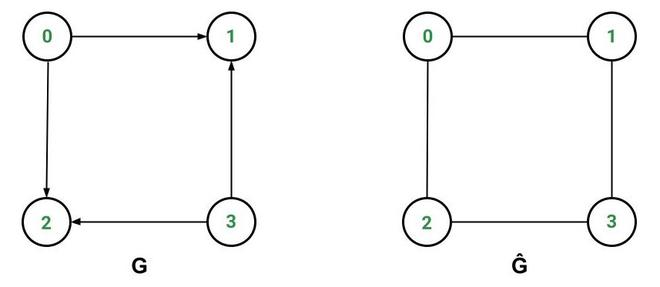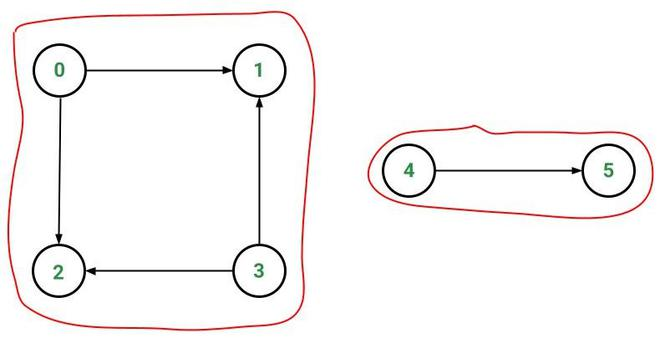# Find Weakly Connected Components in a Directed Graph

• Last Updated : 28 Oct, 2021

Weakly Connected Graph:

A directed graphG = (V, E)’ is weakly connected if the underlying undirected graph Ĝ is connected.

Attention reader! Don’t stop learning now. Get hold of all the important DSA concepts with the DSA Self Paced Course at a student-friendly price and become industry ready.  To complete your preparation from learning a language to DS Algo and many more,  please refer Complete Interview Preparation Course.

In case you wish to attend live classes with experts, please refer DSA Live Classes for Working Professionals and Competitive Programming Live for Students.

The underlying undirected graph is the graph Ĝ = (V, Ê) where Ê represents the set of undirected edges that is obtained by removing the arrowheads from the directed edges and making them bidirectional in G.

Example:The directed graph G above is weakly connected since its underlying undirected graph Ĝ is connected.

Weakly Connected Component:

Given a directed graph, a weakly connected component (WCC) is a subgraph of the original graph where all vertices are connected to each other by some path, ignoring the direction of edges.

Example:In the above directed graph, there are two weakly connected components:

• [0, 1, 2, 3]
• [4, 5]

Algorithm to find Weakly Connected Component:

It uses the algorithm to find connected components of an undirected graph.

• Construct the underlying undirected graph of the given directed graph.
• Find all the connected components of the undirected graph.
• The connected components of the undirected graph will be the weakly connected components of the directed graph.

Implementation:

Below is the code of Weakly Connected Component which takes a directed graph DG as input and returns all the weakly connected components WCC of the input graph.

## Java

 `// Java Code for the above algorithm``import` `java.util.ArrayList;`` ` `class` `Graph {``    ``int` `vertices;``    ``ArrayList > adjacencyList;`` ` `    ``public` `Graph(``int` `vertices)``    ``{``        ``this``.vertices = vertices;``        ``adjacencyList = ``new` `ArrayList<>(vertices);`` ` `        ``for` `(``int` `i = ``0``; i < ``this``.vertices; i++)``            ``adjacencyList.add(``new` `ArrayList<>());``    ``}`` ` `    ``public` `void` `addEdge(``int` `u, ``int` `v)``    ``{``        ``// Use of noEdge(int, int)``        ``// prevents duplication of edges``        ``if` `(noEdge(u, v))``            ``adjacencyList.get(u).add(v);``    ``}`` ` `    ``// Returns true if there does NOT exist``    ``// any edge from u to v``    ``boolean` `noEdge(``int` `u, ``int` `v)``    ``{``        ``for` `(``int` `destination : adjacencyList.get(u))``            ``if` `(destination == v)``                ``return` `false``;``        ``return` `true``;``    ``}``}`` ` `class` `WCC {``    ``private` `final` `Graph directedGraph;`` ` `    ``public` `WCC(Graph directedGraph)``    ``{``        ``this``.directedGraph = directedGraph;``    ``}`` ` `    ``// Finds all the connected components``    ``// of the given undirected graph``    ``private` `ArrayList >``    ``connectedComponents(Graph undirectedGraph)``    ``{``        ``ArrayList > connectedComponents``            ``= ``new` `ArrayList<>();``        ``boolean``[] isVisited``            ``= ``new` `boolean``[undirectedGraph.vertices];`` ` `        ``for` `(``int` `i = ``0``; i < undirectedGraph.vertices; i++) {``            ``if` `(!isVisited[i]) {``                ``ArrayList component``                    ``= ``new` `ArrayList<>();``                ``findConnectedComponent(i, isVisited,``                                       ``component,``                                       ``undirectedGraph);``                ``connectedComponents.add(component);``            ``}``        ``}`` ` `        ``return` `connectedComponents;``    ``}`` ` `    ``// Finds a connected component``    ``// starting from source using DFS``    ``private` `void``    ``findConnectedComponent(``int` `src, ``boolean``[] isVisited,``                           ``ArrayList component,``                           ``Graph undirectedGraph)``    ``{``        ``isVisited[src] = ``true``;``        ``component.add(src);`` ` `        ``for` `(``int` `v :``             ``undirectedGraph.adjacencyList.get(src))``            ``if` `(!isVisited[v])``                ``findConnectedComponent(v, isVisited,``                                       ``component,``                                       ``undirectedGraph);``    ``}`` ` `    ``public` `ArrayList >``    ``weaklyConnectedComponents()``    ``{``        ``// Step 1: Construct the``        ``// underlying undirected graph``        ``Graph undirectedGraph``            ``= ``new` `Graph(directedGraph.vertices);``        ``for` `(``int` `u = ``0``; u < directedGraph.vertices; u++) {``            ``for` `(``int` `v :``                 ``directedGraph.adjacencyList.get(u)) {``                ``undirectedGraph.addEdge(u, v);``                ``undirectedGraph.addEdge(v, u);``            ``}``        ``}`` ` `        ``// Step 2: Find the connected components``        ``// of the undirected graph``        ``return` `connectedComponents(undirectedGraph);``    ``}``}`` ` `public` `class` `WCCDemo {``    ``// Driver Code``    ``public` `static` `void` `main(String[] args)``    ``{``        ``Graph directedGraph = ``new` `Graph(``6``);`` ` `        ``directedGraph.addEdge(``0``, ``1``);``        ``directedGraph.addEdge(``0``, ``2``);``        ``directedGraph.addEdge(``3``, ``1``);``        ``directedGraph.addEdge(``3``, ``2``);``        ``directedGraph.addEdge(``4``, ``5``);`` ` `        ``ArrayList >``            ``weaklyConnectedComponents``            ``= ``new` `WCC(directedGraph)``                  ``.weaklyConnectedComponents();`` ` `        ``int` `index = ``1``;``        ``for` `(ArrayList component :``             ``weaklyConnectedComponents) {``            ``System.out.print(``"Component "` `                             ``+ index++ + ``": "``);``            ``for` `(Integer i : component)``                ``System.out.print(i + ``" "``);``            ``System.out.println();``        ``}``    ``}``}`
Output
```Component 1: 0 1 3 2
Component 2: 4 5 ```

Time complexity: O(V+E)

My Personal Notes arrow_drop_up